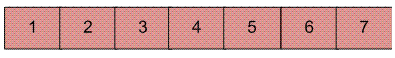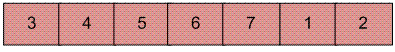# C# Program for Reversal algorithm for array rotation

• Last Updated : 13 Dec, 2021

Write a function rotate(arr[], d, n) that rotates arr[] of size n by d elements.
Example :

```Input :  arr[] = [1, 2, 3, 4, 5, 6, 7]
d = 2
Output : arr[] = [3, 4, 5, 6, 7, 1, 2] ```Rotation of the above array by 2 will make arrayThe first 3 methods to rotate an array by d elements has been discussed in this post.
Method 4 (The Reversal Algorithm) :
Algorithm :

```rotate(arr[], d, n)
reverse(arr[], 1, d) ;
reverse(arr[], d + 1, n);
reverse(arr[], 1, n);```

Let AB are the two parts of the input array where A = arr[0..d-1] and B = arr[d..n-1]. The idea of the algorithm is :

• Reverse A to get ArB, where Ar is reverse of A.
• Reverse B to get ArBr, where Br is reverse of B.
• Reverse all to get (ArBr) r = BA.

Example :
Let the array be arr[] = [1, 2, 3, 4, 5, 6, 7], d =2 and n = 7
A = [1, 2] and B = [3, 4, 5, 6, 7]

• Reverse A, we get ArB = [2, 1, 3, 4, 5, 6, 7]
• Reverse B, we get ArBr = [2, 1, 7, 6, 5, 4, 3]
• Reverse all, we get (ArBr)r = [3, 4, 5, 6, 7, 1, 2]

Below is the implementation of the above approach :

## C#

 `// C# program for reversal algorithm``// of array rotation``using` `System;`` ` `class` `GFG {``    ``/* Function to left rotate arr[]``    ``of size n by d */``    ``static` `void` `leftRotate(``int``[] arr, ``int` `d)``    ``{`` ` `        ``if` `(d == 0)``            ``return``;``        ``int` `n = arr.Length;``          ``// in case the rotating factor is``        ``// greater than array length``        ``d = d % n;``        ``reverseArray(arr, 0, d - 1);``        ``reverseArray(arr, d, n - 1);``        ``reverseArray(arr, 0, n - 1);``    ``}`` ` `    ``/* Function to reverse arr[] from``    ``index start to end*/``    ``static` `void` `reverseArray(``int``[] arr, ``int` `start,``                             ``int` `end)``    ``{``        ``int` `temp;``        ``while` `(start < end) {``            ``temp = arr[start];``            ``arr[start] = arr[end];``            ``arr[end] = temp;``            ``start++;``            ``end--;``        ``}``    ``}`` ` `    ``/*UTILITY FUNCTIONS*/``    ``/* function to print an array */``    ``static` `void` `printArray(``int``[] arr)``    ``{``        ``for` `(``int` `i = 0; i < arr.Length; i++)``            ``Console.Write(arr[i] + ``" "``);``    ``}`` ` `    ``// Driver code``    ``public` `static` `void` `Main()``    ``{``        ``int``[] arr = { 1, 2, 3, 4, 5, 6, 7 };``        ``int` `n = arr.Length;``        ``int` `d = 2;`` ` `        ``leftRotate(arr, d); ``// Rotate array by 2``        ``printArray(arr);``    ``}``}`` ` `// This code is contributed by Sam007`

Output :

`3 4 5 6 7 1 2`

Time Complexity : O(n)

Please refer complete article on Reversal algorithm for array rotation for more details!

My Personal Notes arrow_drop_up